PRINTABLE FOR KIDS

XII (12) HSC

XI (11) FYJC
X (10) SSC

### Problem Set 3 Polynomials Class 9th Mathematics Part I MHB Solution

Problem Set 3 Polynomials Class 9th Mathematics Part I MHB Solution

###### Problem Set 3
Question 1.

Write the correct alternative answer for each of the following questions.

Which of the following is a polynomial?
A. x/y

B. x√2 – 3x

C. x-2 + 7

D.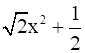Option (A)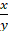is not a polynomial. Hence, incorrect option.

(B) is x√2 – 3x is not a polynomial because it does not have natural number power. Hence, incorrect.

(C) x-2 + 7 is not a polynomial. Because it has negative power. Hence, incorrect.

(D)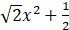is the quadratic polynomial.

The option D is the only valid polynomial because in this polynomial only the power of the variable is a whole number.

Question 2.

Write the correct alternative answer for each of the following questions.

What is the degree of the polynomial √7?
A. 1/2

B. 5

C. 2

D. 0

The degree of this polynomial is zero as √7x0.

Option (A) is not the correct polynomial as a degree of polynomial cannot be fractional.

Option (B) is also not the correct answer as √7 is not multiplied with a variable with degree 5.

Option (C) is also not the correct answer as √7 is not multiplied with a variable with degree.

Option (D) is the correct answer as x0 is multiplied with √7

The degree of this polynomial is zero as √7x0.

Question 3.

Write the correct alternative answer for each of the following questions.

What is the degree of the 0 polynomial?
A. 0

B. 1

C. undefined

D. any real number

Option (A) is not the degree of zero polynomial as zero polynomial does not have any variable and so its degree cannot be zero.

Option (B) is not the degree of zero polynomial as zero polynomial does not have any variable and so its degree cannot be one.

Option (C) is the degree of zero polynomial as zero polynomial does not have any variable and so its degree is not defined.

Option (D) is not the degree of zero polynomial as zero polynomial does not have any variable and so its degree is not defined and hence the degree cannot be any real number.

So the correct answer is C.

Question 4.

Write the correct alternative answer for each of the following questions.

What is the degree of the polynomial 2x2 + 5x3 + 7?
A. 3

B. 2

C. 5

D. 7

The degree of a polynomial is the highest degree of its monomials (individual terms) with non-zero coefficients.

Therefore, the degree of this polynomial is 3

Option (B) is not correct as the degree does not match to the highest degree of the variable.

Option (C) is not correct as the degree does not match to the highest degree of the variable.

Option (D) is not correct as the degree does not match to the highest degree of the variable.

So option A is correct.

Question 5.

Write the correct alternative answer for each of the following questions.

What is the coefficient form of x3 – 1?
A. (1, - 1)

B. (3, - 1)

C. (1, 0,0, -1)

D. (1, 3, - 1)

The coefficient form is as follows:

x3 – 1 = x3 + 0x2 + 0x – 1

The coefficient form is:

(1, 0, 0, -1)

Option (A) does not match to our solution.

Option (B) does not match to our solution.

Option (C) does match to our solution.

Option (D) also does not match to our solution.

So option (C) is correct answer.

Question 6.

Write the correct alternative answer for each of the following questions.

p(x) = x2 - 7√7 + 3 then p(7√7)=?
A. 3

B. 7√7

C. 42√7 + 3

D. 49√7

Put p =7√7 throughout the polynomial

= (7√7)2 – (7√7 × 7√7) + 3

=49 × 7 – (49 × 7) + 3

=343 – 343 + 3

=3

Option (A) does match to our solution.

Option (B) does not match to our solution.

Option (C) does not match to our solution.

Option (D) also does not match to our solution.

So option (A) is correct answer.

Question 7.

Write the correct alternative answer for each of the following questions.

When x= -1, what is the value of the polynomial 2x3 + 2x?
A. 4

B. 2

C. - 2

D. – 4

Put x = -1 throughout the polynomial

= 2 × (-1)3 + (2 × -1)

= -2 -2

= -4

Option (A) does not match to our solution.

Option (B) does not match to our solution.

Option (C) does not match to our solution.

Option (D) does match to our solution.

So option (D) is correct answer.

Question 8.

Write the correct alternative answer for each of the following questions.

If x – 1, what is a factor of the polynomial 3x2 + mx then find the value of m.
A. 2

B. - 2

C. - 3

D. 3

Put x = 1 in the given polynomial

3 × 1 + m = 0

m = -3

Option (A) does not match to our solution.

Option (B) does not match to our solution.

Option (C) does match to our solution.

Option (D) also does not match to our solution.

So option (C) is correct answer.

Question 9.

Write the correct alternative answer for each of the following questions.

Multiply (x2 -3) × (2x – 7x3 + 4) and write the degree of the product.
A. 5

B. 3

C. 2

D. 0

The multiplication is as follows:

= (x2 -3) × (2x – 7x3 + 4)

= x2.2x – 7x3.x2 + 4x2 -3.2x + 3.7x3 -3.4

= 2.x2+1 – 7x3+2 + 4x2 -6x + 21x3-12

= 2x3 – 7x5 + 4x2 – 6x + 21x3 – 12

“.” represents Multiplication

Therefore the degree of the product is 5.

Option (B) is not correct as the degree does not match to the highest degree of the variable.

Option (C) is not correct as the degree does not match to the highest degree of the variable.

Option (D) is not correct as the degree does not match to the highest degree of the variable.

So option A is correct.

Question 10.

Write the correct alternative answer for each of the following questions.

Which of the following is a linear polynomial?
A. x + 5

B. x2 + 5

C. x3 + 5

D. x4 + 5

A polynomial is said to be linear if the highest power of the variable is one. So among the options x + 5 only has highest power as one.

Option (B) is not correct as the highest degree of the variable is 2 and the equation is quadratic.

Option (C) is not correct as the highest degree of the variable is 3 and the equation is cubic.

Option (D) is not correct as the highest degree of the variable is 4.

So option A is correct.

Question 11.

Write the degree of the polynomial for each of the following.

i. 5 + 3x4

ii. 7

iii. ax7 + bx9 (a, b are constants)

i. The degree of a polynomial is the highest degree of its monomials (individual terms) with non-zero coefficients.
Here,

3x4 + 0.x3 + 0.x2 + 0.x + 5

Therefore, the degree of this polynomial is 4

ii. The degree of a polynomial is the highest degree of its monomials (individual terms) with non-zero coefficients.

Here,

7x0.

Therefore, the degree of this polynomial is 0

iii. The degree of a polynomial is the highest degree of its monomials (individual terms) with non-zero coefficients.

Here,

b.x9 + 0.x8 + a.x7 + 0.x6 + 0.x5 + 0.x4 + 0.x3 + 0.x2 + 0.x1 + 1

Therefore, the degree of this polynomial is 9.

Question 12.

Write the following polynomials in standard form.

i. 4x2 + 7x4 - x3 – x - 9

ii. p + 2p3 + 10p2 + 5p4 – 8

i. In standard form the terms are arranged from highest power of the variable to the lowest power.

So the standard form is as follows:

7x4 – x3 + 4x2 – x + 9.

ii. In standard form the terms are arranged from highest power of the variable to the lowest power.

So the standard form is as follows:

5p4 + 2p3 + 10p2 + p – 8.

Question 13.

Write the following polynomial in coefficient form.

i. x4 + 16

ii. m5 + 2m2 + 3m + 15

i. In the coefficient form of the polynomial, the coefficients of the terms are written from highest power of the variable to lowest power.

⟹ x4 + 16 = 1.x4 + 0.x3 + 0.x2 + 0.x + 16

So the coefficient form is as follows:

(1, 0, 0, 0, 16).

ii. In the coefficient form of the polynomial, the coefficients of the terms are written from highest power of the variable to lowest power.

⟹ m5 + 2m2 + 3m + 15 = 1.m5 + 0.m4 +0.m3 + 2.m2 + 3.m + 15

So the coefficient form is as follows:

(1, 0, 0, 2, 3, 15).

Question 14.

Write the index form of the polynomial using variable x from its coefficient form.

i. (3, -2, 0, 7, 18)

ii. (6, 1, 0, 7)

iii. (4, 5, -3, 0)

i. The given representation is the coefficient form of the polynomial. So, the first coefficient denotes the highest power of the variable and the power of last term is always zero.

So the index form is as follows:

3x4 – 2x3 + 0x2 + 7x + 18

= 3x4 – 2x3 + 7x + 18

ii. The given representation is the coefficient form of the polynomial. So, the first coefficient denotes the highest power of the variable and the power of last term is always zero.

So the index form is as follows:

6x3 + x2 + 0x + 7

=6x3 + x2 + 7

iii. The given representation is the coefficient form of the polynomial. So, the first coefficient denotes the highest power of the variable and the power of last term is always zero.

So the index form is as follows:

4x3 + 5x2 -3x + 0

= 4x3 + 5x2 -3x

Question 15.

Add the following polynomials.

7x4 – 2x3 + x 10; 3x4 + 15x3 + 9x2 – 8x + 2

The addition is as follows: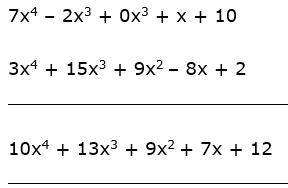The result is as follows: 10x4 + 13x3 + 9x2 + 7x + 12

Question 16.

Add the following polynomials.

3p3q + 2p2q + 7; 2p2q + 4pq – 2p3q

The addition is as follows: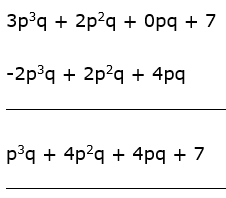The result is as follows: p3q + 4p2q + 4pq + 7

Question 17.

Subtract the second polynomial from the first.

5x2 – 2y + 9; 3x2 + 5y - 7

The subtraction is as follows: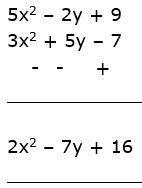The result is as follows: 2x2 – 7y + 16

Question 18.

Subtract the second polynomial from the first.

2x2 + 3x + 5; x2 – 2x + 3

The subtraction is as follows: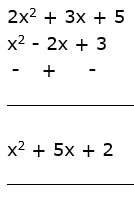The result is as follows: x2 + 5x + 2

Question 19.

Multiply the following polynomials.

(m3 – 2m + 3) (m4 -2m2 + 3m + 2)

The multiplication is as follows:

= (m3 – 2m + 3) × (m4 -2m2 + 3m + 2)

= m× m4 - 2m× m3 + 3m × m3 + 2 × m3 - 2m × m4 + 4m× m – 3 × 2m - 4m + 3m4 – 6m2 + 6m + 12

= m7 – 2m5 + 3m4 + 2m3 – 2m5 + 4m3 – 6m - 4m + 3m4 - 6m2 + 6m + 12

= m7 - 4m5 + 6m4 + 6m3 – 16m + 12

The product = m7 - 4m5 + 6m4 + 6m3 – 16m + 12

Question 20.

Multiply the following polynomials.

(5m3 – 2) (m2 –m +3)

The multiplication is as follows:

= (5m3 – 2) × (m2 –m +3)

= 5m3.m2 – 5m3.m + 3.5m3 – 2m2 + 2m – 6

= 5m5 – 5m4 + 15m3 – 2m2 + 2m -6

The product is = 5m5 – 5m4 + 15m3 – 2m2 + 2m -6

Question 21.

Divide polynomial 3x3 – 8x2 + x + 7 by x – 3 using synthetic method and write the quotient and remainder.

The coefficient forms the first polynomial is as follows:

⟹ 3x3 – 8x2 + x + 7

⟹ (3, -8, 1, 7)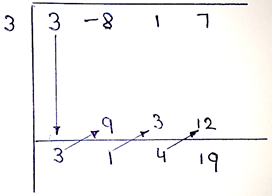The quotient is as follows = 3x3 + x2 + 4x

The remainder is = 19

Question 22.

For which the value of x + 3 is the factor of the polynomial x3 – 2mx + 21?

Put x = -3 in the given polynomial and equate it to zero as x+3 is to be a factor of the polynomial.

(-3)3 – 2 × m × (-3) + 21 = 0

-27 + 6m + 21 = 0

6m -6 = 0

6m = 6

m = 1

So for x+3 to be a factor of the given polynomial, the value of m = 1.

Question 23.

At the end of the year 2016, the population of villages Kovad, Varud, Chikhali is 5x2 – 3y2, 7y2 + 2xy and 9x2 + 4xy respectively. At the beginning of the year 2017, x2 + xy – y2,5xy and 3x2 + xy persons from each of the three villages respectively went to another village for education then what is the remaining total population of these three villages?

Older Population of Kovad, Pk = 5x2 – 3y2

Older Population of Varud, Pv = 7y2 + 2xy

Older Population of Chikhali, Pc = 9x2 + 4xy

Number of people migrated from Kovad, Mk = x2 + xy – y2

Number of people migrated from Varud, Mv = 5xy

Number of people migrated from Chikhali, Mc = 3x2 + xy

Present Population of Kovad = Pk – Mk

= (5x2 – 3y2) – (x2 + xy – y2)

= 4x2 – 2y2 – xy

Present population of Varud = Pv – Mv

= (7y2 + 2xy) – 5xy

= 7y2 – 3xy

Present Population of Chikhali = Pc – Mc

= (9x2 + 4xy) – (3x2 + xy)

= 6x2 + 3xy

Question 24.

Polynomials bx2 + x + 5 and bx3 – 2x + 5 are divided by polynomial x – 3 and the remainders are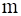and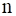respectively. If m – n =0 then find the value of b.

Put x = 3 in the first polynomial:

b× (3)2 + 3 + 5 = m

9b + 8 = m ………………………………….. (i)

Put x = 3 in the second polynomial

b× (3)3 -2× 3 + 5 = n

27b -6 + 5 = n

27b -1 = n …………………………………. (ii)

Equation (i) – (ii)

m –n = 9b + 8 – (27b -1)

but m-n = 0

9b + 8 -27b + 1 = 0

-18b + 9 = 0

18b = 9

b = 9/18

b = 1/2

Question 25.

Simplify.

(8m2 + 3m – 6) - (9m-7) + (3m2 – 2m + 4)

(8m2 + 3m – 6) - (9m-7) + (3m2 – 2m + 4)

= 8m2 + 3m – 6 – 9m + 7 + 3m2 – 2m + 4

= 11m2 – 8m + 5

Question 26.

Which polynomial is to be subtracted from x2 + 13x + 7 to get the polynomial 3x2 + 5x – 4?

Let the polynomial to be subtracted be ax2 + bx + c

3x2 + 5x – 4 = (x2 + 13x + 7) - (ax2 + bx + c)

(ax2 + bx + c) = (x2 + 13x + 7) – (3x2 + 5x – 4)

= (x2 – 3x2) + (13x – 5x) + (7 + 4)

= -2x2 – 8x + 11

Comparing with coefficients we get:

a= -2

b = -8

c = 11

Therefore, the polynomial is = -2x2 -8x + 11

Question 27.

Which polynomial is to be added to 4m + 2n + 3 to get the polynomial 6m + 3n + 10?

Let the polynomial to be added be am + bn + c

6m + 3n + 10 = (4m + 2n + 3) + (am + bn + c)

(am + bn + c) = (6m + 3n + 10) - (4m + 2n + 3)

= (6-4) m + (3-2) n + (10-3)

= 2m + n + 7

Therefore, the polynomial to be added to 4m + 2n + 3 is 2m + n + 7.

## PDF FILE TO YOUR EMAIL IMMEDIATELY PURCHASE NOTES & PAPER SOLUTION. @ Rs. 50/- each (GST extra)

SUBJECTS

HINDI ENTIRE PAPER SOLUTION

MARATHI PAPER SOLUTION
SSC MATHS I PAPER SOLUTION
SSC MATHS II PAPER SOLUTION
SSC SCIENCE I PAPER SOLUTION
SSC SCIENCE II PAPER SOLUTION
SSC ENGLISH PAPER SOLUTION
SSC & HSC ENGLISH WRITING SKILL
HSC ACCOUNTS NOTES
HSC OCM NOTES
HSC ECONOMICS NOTES
HSC SECRETARIAL PRACTICE NOTES

2019 Board Paper Solution

HSC ENGLISH SET A 2019 21st February, 2019

HSC ENGLISH SET B 2019 21st February, 2019

HSC ENGLISH SET C 2019 21st February, 2019

HSC ENGLISH SET D 2019 21st February, 2019

SECRETARIAL PRACTICE (S.P) 2019 25th February, 2019

HSC XII PHYSICS 2019 25th February, 2019

CHEMISTRY XII HSC SOLUTION 27th, February, 2019

OCM PAPER SOLUTION 2019 27th, February, 2019

HSC MATHS PAPER SOLUTION COMMERCE, 2nd March, 2019

HSC MATHS PAPER SOLUTION SCIENCE 2nd, March, 2019

SSC ENGLISH STD 10 5TH MARCH, 2019.

HSC XII ACCOUNTS 2019 6th March, 2019

HSC XII BIOLOGY 2019 6TH March, 2019

HSC XII ECONOMICS 9Th March 2019

SSC Maths I March 2019 Solution 10th Standard11th, March, 2019

SSC MATHS II MARCH 2019 SOLUTION 10TH STD.13th March, 2019

SSC SCIENCE I MARCH 2019 SOLUTION 10TH STD. 15th March, 2019.

SSC SCIENCE II MARCH 2019 SOLUTION 10TH STD. 18th March, 2019.

SSC SOCIAL SCIENCE I MARCH 2019 SOLUTION20th March, 2019

SSC SOCIAL SCIENCE II MARCH 2019 SOLUTION, 22nd March, 2019

XII CBSE - BOARD - MARCH - 2019 ENGLISH - QP + SOLUTIONS, 2nd March, 2019

HSC Maharashtra Board Papers 2020

(Std 12th English Medium)

HSC ECONOMICS MARCH 2020

HSC OCM MARCH 2020

HSC ACCOUNTS MARCH 2020

HSC S.P. MARCH 2020

HSC ENGLISH MARCH 2020

HSC HINDI MARCH 2020

HSC MARATHI MARCH 2020

HSC MATHS MARCH 2020

SSC Maharashtra Board Papers 2020

(Std 10th English Medium)

English MARCH 2020

HindI MARCH 2020

Hindi (Composite) MARCH 2020

Marathi MARCH 2020

Mathematics (Paper 1) MARCH 2020

Mathematics (Paper 2) MARCH 2020

Sanskrit MARCH 2020

Important-formula

THANKS